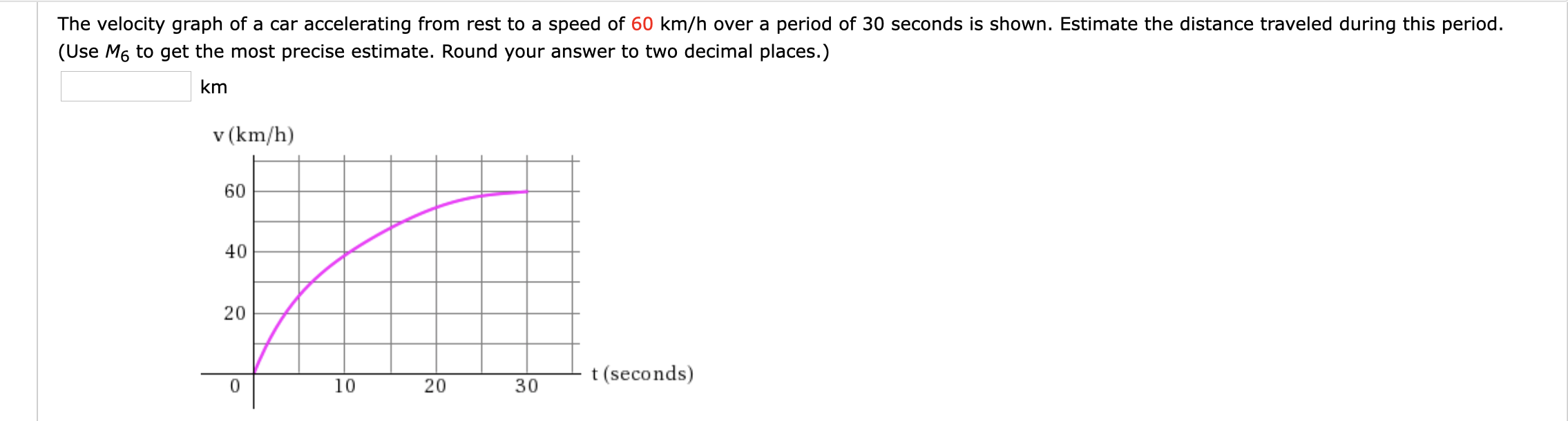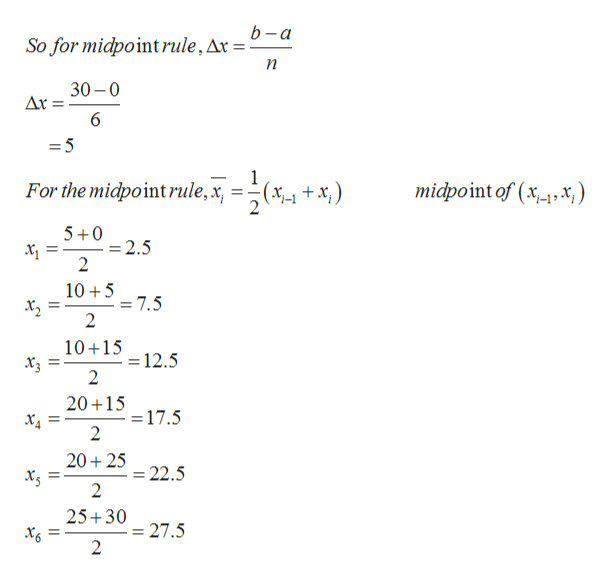# The velocity graph of a car accelerating from rest to a speed of 60 km/h over a period of 30 seconds is shown. Estimate the distance traveled during this period.(Use M6 to get the most precise estimate. Round your answer to two decimal places.)kmv (km/h)604020t(seconds)0103020

Question
1991 viewshelp_outlineImage TranscriptioncloseThe velocity graph of a car accelerating from rest to a speed of 60 km/h over a period of 30 seconds is shown. Estimate the distance traveled during this period. (Use M6 to get the most precise estimate. Round your answer to two decimal places.) km v (km/h) 60 40 20 t(seconds) 0 10 30 20 fullscreen
check_circle

star
star
star
star
star
1 Rating
Step 1

The velocity graph of a car accelerating from rest to a speed of 60 km/h over a period of 30 seconds is shown. Estimate the distance, d traveled during this period.

Step 2

Here M6 rule means use midpoint rule with n=6
Here the range is 0 to 30help_outlineImage Transcriptioncloseb-a So for midpointrule,Ar =- п 30 0 Ar 6 =5 1 For the midpoint rule,x midpoint of (x,x,) (x- +x,) 5 0 =2.5 2 105 = 7.5 2 10+15 =12.5 2 20+15 = 17.5 2 20 25 = 22.5 2 25+30 = 27.5 2 X6 = fullscreen
Step 3

Now estimate y values at those x values

Roughly they are 15,32,45,52,an...

### Want to see the full answer?

See Solution

#### Want to see this answer and more?

Solutions are written by subject experts who are available 24/7. Questions are typically answered within 1 hour.*

See Solution
*Response times may vary by subject and question.
Tagged in

### Other